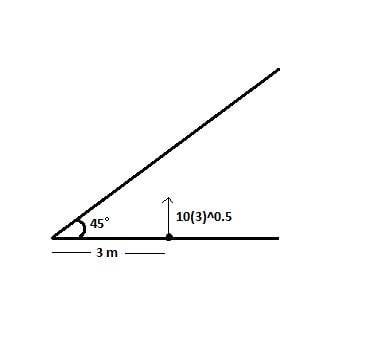# Inelastic collision with an inclined plane

## Homework Statement

A particle is thrown upward with speed 10√3 m/sec. It strikes an inclined surface. Collision between the particle and inclined plane is perfectly inelastic. What will be the maximum height attained by the particle from the ground.2. Relevant information and equations
• In perfectly inelastic collision (e=0) colliding objects stick to each other.
• When net F external = 0, momentum is conserved.
• v2 = u2 + 2as (for constant acceleration a)

## The Attempt at a Solution

Just before collision let velocity of particle be v
⇒ v2 = (10√3)2 - 2g(3)
⇒ v = 4√15 m/s (g=10 m/s2

Let m = mass of particle, M = mass of surface, V = velocity of particle and surface along the normal.

Along the normal,
∫Ndt = m(V-vcos45°) = MV
⇒V = mv/√2(m-M)
Since denominator is very large, V≈ 0

Now component of velocity along the inclined plane ( = vsin45°) will not change as the normal reaction (which will give the impulse during collision) is acting to perpendicular to it.

So finally particle will move along the incline particle( upwards) till its velocity becomes zero.
0 = (vsin45°)2 - 2s(gsin45°)
⇒ s = 6√2

Thus, total height above ground = s(sin45°) + 3 = 9 m
But the answer is 6 m.
Is it because I neglected the velocity along the normal?

#### Attachments

haruspex
Homework Helper
Gold Member
2020 Award
In perfectly inelastic collision (e=0) colliding objects stick to each other.
That is only true for head on collisions.
So finally particle will move along the incline
It will move that way immediately after impact, but there is no glue holding it to the ceiling.

That is only true for head on collisions.
But they will still move together along the normal, right? Because velocity of separation = 0
It will move that way immediately after impact, but there is no glue holding it to the ceiling
So, will it be like a projectile?
I don't understand how that's possible if the surface and ball are moving together along normal. What does perfectly inelastic collision signify here?

haruspex
Homework Helper
Gold Member
2020 Award
But they will still move together along the normal, right?
Only at first.
So, will it be like a projectile?
Yes.
What does perfectly inelastic collision signify here?
That immediately after collision the velocities are the same in the direction of the impulse, so zero in this case.
Gravity continues to act, and its component in the direction of impulse is away from the ceiling, so the particle will separate from it.

Only at first.
Okay I get it now.
At maximum height, velocity along y axis = 0
⇒ 0 = (v/2)2 - 2gs
⇒ s = 3 m
Total height above ground = 3 +3 = 6 m
Thanks you for helping me.haruspex
Homework Helper
Gold Member
2020 Award
Okay I get it now.
At maximum height, velocity along y axis = 0
⇒ 0 = (v/2)2 - 2gs
⇒ s = 3 m
Total height above ground = 3 +3 = 6 m
Thanks you for helping me.You are welcome.

Orodruin
Staff Emeritus
•